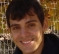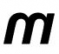## Efficient way to adjust the magnitude of a complex numberStarted by 6 years ago10 replieslatest reply 6 years ago229 views

I am doing some spectral subtraction type DSP and I have found that one of the major sources of computation is the adjustment(via subtraction) of the complex frame values. The operation I am interested in is: given a complex number, x, then set it's magnitude to d, i.e.

y = x / abs(x) * d

In my particular case I already know abs(x) and I am working with 32 bit integer arithmetic.

My question is: are there any tricks (avoiding divides, etc) that are known for this operation? Thanks

[ - ]In FPGAs we sometimes resort to lookup tables(LUT) for all or part of equations at the expense of memory.

In your case you can do all in LUT i.e.

you use re(x) and image(x) as address. The content of each location will be value of

re(x)/abs(x) * d & im(x)/abs(x) * d  assuming d is constant else you can use multiplier for d

Or just 1/abs(x) as content to be multiplied by re(x),im(x)

The LUTs can be very large or you can opt for small LUT + some interpolation

[ - ]That's a nice idea. I'll model the error and see how much interpolation i can get away with as a massive LUT is not preferable but the speed it offers is.

I assume a 2D LUT would be in order. And i could reduce memory by removing symmetries from it.

Thank you

[ - ]Actually I added to above post:

You may just use 1/abs(x) as precomputed content to be multiplied at run time by re(x),im(x) & d

[ - ]Thanks, that's even better.

[ - ]To reduce LUT length, use abs(x) as LUT address, instead of re(x) and image(x).

If you want to further reduce LUT, you can convert abs(x)=M*2^E, where M is a short mantissa and E is the exponent. Them you use M as address for a LUT to obtain G=d/M, and apply a gain of y = (x*G)>>E.

[ - ]What kind of processor are you using?  To calculate abs(x) you need a pair of multiplies and an addition. If x is complex the divide is actually two divides. I would think that using a part with a divide instruction (Cortex M3 or M4, for example) it would be faster to just use divides than a lookup table or pair of lookup tables

[ - ]I'm using an xcore processor. On which divides can be about 10 times the cost of a multiply from a LUT.

[ - ]Snap!  Fair enough

[ - ]